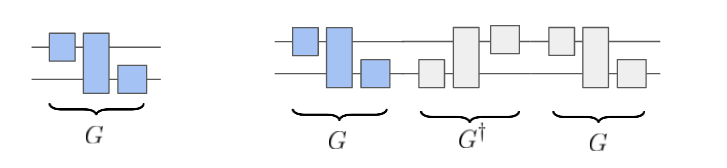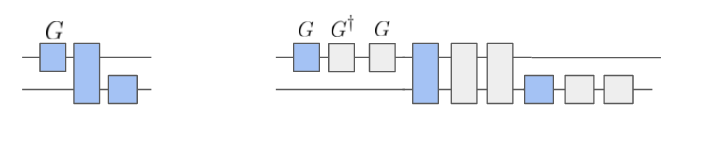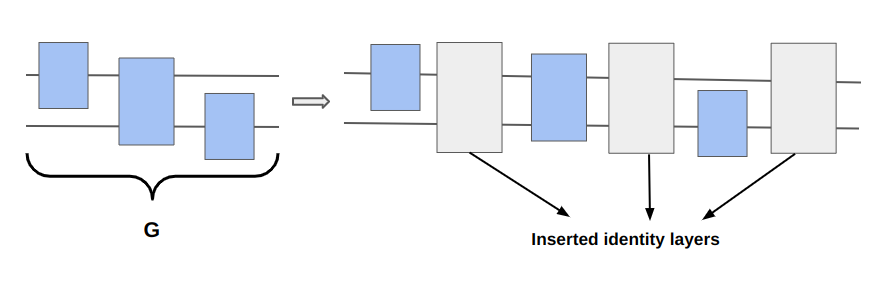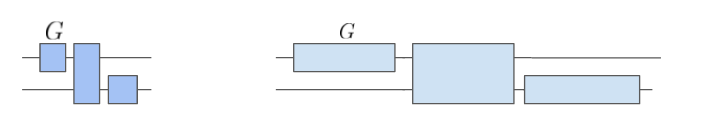# What is the theory behind ZNE?#

Zero noise extrapolation (ZNE) [4, 5, 6] is an error mitigation technique used to extrapolate the noiseless expectation value of an observable from a range of expectation values computed at different noise levels. This process works in two steps:

• Step 1: Intentionally scale noise. This can be done with different methods. Pulse-stretching  can be used to increase the noise level of a quantum computation. Similar results can be obtained, at a gate-level, with unitary folding [4, 7] or identity insertion scaling.

• Step 2: Extrapolate to the noiseless limit. This can be done by fitting a curve (often called extrapolation model) to the expectation values measured at different noise levels to extrapolate the noiseless expectation value.

## Step 1: Intentionally scale noise.#

A technique to increase the noise level of a circuit at the gate level is to intentionally increase its depth. This can be obtained using either unitary folding or identity scaling.

In unitary folding, we perform a mapping $$G \mapsto G G^\dagger G$$. This mapping can be applied globally or locally as shown in the diagrams below.The diagram demonstrates how gates are inserted in a circuit when global folding is applied.#The diagram demonstrates how gates are inserted in a circuit when local folding is applied.#

More details on the theory of unitary folding can be found in .

In identity insertion scaling, we perform a mapping $$G \mapsto I G$$. This mapping can be applied as shown in the diagram below.The diagram demonstrates how identity gates are inserted after a circuit layer when identity insertion scaling function is applied.#

Additional details on the theory of identity insertion scaling are similar to those in unitary folding. The only difference is that instead of scaling gate noise, the insertion of an identity gate increases the wait time after each circuit layer is executed. This allows the qubits to interact with the environment through some noisy process and decohere if the system-environment interaction is strong. The decoherence time for the qubits in a quantum system is determined by the amount of time our system of interest remains coherent and uncouples from the external environment. More details on decoherence can be found in [8, 9].

More details on practical implementation of both methods in Mitiq can be found in What additional options are available for ZNE?.

A noise scaling technique similar to unitary folding is pulse-stretching: a method that only applies to devices with pulse-level access [5, 6]. The noise of the device can be altered by increasing the time over which pulses are implemented, as shown in the following diagram:The diagram demonstrates how pulse stretching is used to increase noise in a physical device for ZNE.#

## Step 2: Extrapolate to the noiseless limit#

In both gate-model and pulse-model scenarios, let $$\tau$$ be a parameter quantifying noise level in the circuit and let $$\tau^\prime = \lambda \tau$$ the scaled noise level.

For $$\lambda = 1$$, the input circuit remains unchanged since the noise level $$\tau^\prime = \tau$$ is the same as the noise of the physical device without any scaling.

Let $$\rho(\tau')$$ be the state prepared by a noise scaled quantum circuit. The expectation value of an observable $$A$$ can be described as a function of the noise scaling parameter as follows:

$\langle E(\lambda) \rangle = \text{Tr}[\rho(\tau') A] = \text{Tr}[\rho(\lambda \tau) A]$

The idea of ZNE is that one can estimate the ideal expectation value $$\langle E(\lambda=0) \rangle$$, by measuring a range of different expectation values $$\langle E(\lambda) \rangle$$ for different values of $$\lambda \ge 1$$ and extrapolating to the zero-noise limit.

In practice the extrapolation can be done as follows:

1. Assume $$E(\lambda)\simeq f(\lambda; p_1, p_2, ... p_m)$$, where $$f$$ is an extrapolation model, i.e., a function of $$\lambda$$ depending on a set of real parameters $$p_1, p_2, \dots, p_m$$.

2. Fit the function $$f$$ to the measured noise-scaled expectation values, obtaining an optimal set of parameters $$\tilde p_1, \tilde p_2, \dots \tilde p_m$$.

3. Evaluate the corresponding zero-noise limit, i.e., $$f(0; \tilde p_1, \tilde p_2, \dots \tilde p_m)$$.

Different choices of $$f$$, produce different extrapolations. Typical choices for $$f$$ are: a linear function, a polynomial, an exponential. For example, Richardson extrapolation, corresponds to the following polynomial model:

$f(\lambda; p_1, p_2, ... p_m) = p_1 + p_2 \lambda + p_3 \lambda^2 + \dots p_m \lambda^{m-1},$

where $$m$$ is equal to the number of data points in the fit (i.e. the number of noise scaled expectation values).

More details on how to apply different extrapolation methods with Mitiq can be found in What additional options are available in ZNE?.